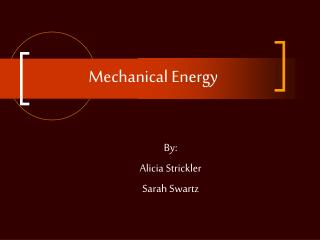DownloadDownload PresentationMechanical Energy

# Mechanical Energy

Download Presentation## Mechanical Energy

- - - - - - - - - - - - - - - - - - - - - - - - - - - E N D - - - - - - - - - - - - - - - - - - - - - - - - - - -
##### Presentation Transcript

1. Mechanical Energy By: Alicia Strickler Sarah Swartz

2. Table Of Contents • Overview of energy • Definition of mechanical energy • Explanation and sources • Real life examples • Pictures • How energy converts

3. Overview Of Energy Energy is the ability to do work. Energy is transferred by a force moving an object through a distance. It is measured in joules.

4. Definition Of Mechanical Energy Mechanical energy is the sum of an objects potential energy and kinetic energy. Mechanical energy is associated with the motion and position of every day objects.

5. Calculating Mechanical Energy Total Mechanical Energy (TME) = PE + KE PE = Potential Energy and KE = Kinetic Energy

6. Explanation And Sources It is the energy which is possessed by an object due to its motion or position. Mechanical energy

7. Kinetic Energy Kinetic energy is the energy of motion. The kinetic energy of any moving object depends upon its mass and speed. Kinetic energy (KE) = 1/2mv² m = mass in kilograms and v = speed in meters per second

8. Examples of kinetic energy

9. Potential Energy Potential energy is energy that is stored as a result of position or shape.

10. Gravitational Potential Energy Gravitational potential energy is potential energy that depends upon an objects height. This energy increases when an object is raised to a higher level. It depends on mass, height, and the acceleration due to gravity. Potential Energy (PE) = mgh Mg = weight in kilograms and H = height in meters

11. Examples Of Potential Energy She has gravitational potential energy by standing on the end of the diving board

12. Other Real Life Examples

13. Other Real Life Examples A moving car A moving baseball

14. Converting Energy Energy conversion is the process of changing energy from one form to another. The law of conservation of energy says energy cannot be created or destroyed. This means it must be converted.

15. Converting Mechanical Energy Mechanical energy is converted to thermal energy when you bounce a ball. When the ball hits the ground, it causes it to heat up.

16. Works Cited Henderson, Tom. "The Physics Classroom Tutorial." 1996 14 Oct 2008 <http://www.glenbrook.k12.il.us/gbssci/phys/Class/energy/u5l1d.html>.